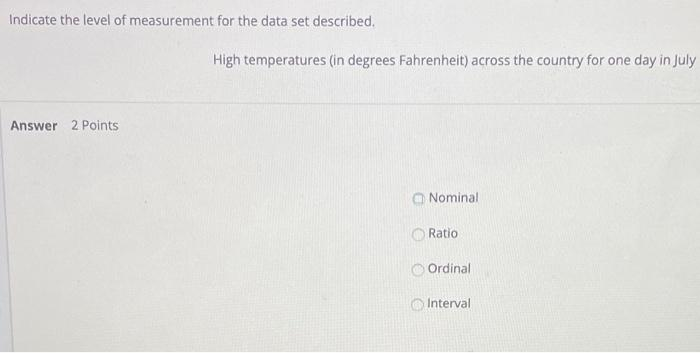# Question Solved1 AnswerIndicate the level of measurement for the data set described. High temperatures (in degrees Fahrenheit) across the country for one day in July Answer 2 Points Nominal Ratio Ordinal IntervalH4YCKF The Asker · Probability and StatisticsTranscribed Image Text: Indicate the level of measurement for the data set described. High temperatures (in degrees Fahrenheit) across the country for one day in July Answer 2 Points Nominal Ratio Ordinal Interval
More
Transcribed Image Text: Indicate the level of measurement for the data set described. High temperatures (in degrees Fahrenheit) across the country for one day in July Answer 2 Points Nominal Ratio Ordinal Interval